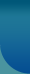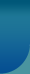Home >> Research Methods and Statistics >> Hypothesis

## Hypothesis

Facts are dependent upon a theoretical framework for their meaning. They are also statements of relationships between concepts. Theory can give direction to the search for facts. A hypothesis states what we are looking for. When facts are assembled, ordered and seen in a relationship they constitute a theory. The theory is not speculation but is built upon fact. Now the various facts in a theory may be logically analyzed and relationships other than those stated in the theory can be deduced. At this point there is no knowledge as to whether such deductions are correct. The formulation of the deduction however constitutes a hypothesis; if verified it becomes part of a future theoretical construction. The relation between the hypothesis and theory is very close indeed. A theory states a logical relationship between facts. From this theory other propositions can be deduced that should be true, if the first relationship holds. These deduced propositions are hypotheses.

A hypothesis looks forward. It is a proposition which can be put to a test to determine its validity. It may seem contrary to or in accord with common sense. It may prove to be correct or incorrect. In any event however, it leads to an empirical test. Whatever the outcome, the hypothesis is a question put in such a way that an answer of some kind can be forthcoming. It is an example of the organized skepticism of science. The refusal to accept any statement without empirical verification. Every worthwhile theory then permits the formulation of additional hypotheses. These when tested are either proved or disapproved and in turn constitute further tests of the original theory.

### Design of Proof: Testing the Hypothesis

The function of the hypothesis is to state a specific relationship between phenomena in such a way that this relationship can be empirically tested. The basic method of this demonstration is to design the research so that logic will require the acceptance or rejection of the hypothesis on the basis of resulting data.

The basic designs of logical proof were formulated by John Stuart Mill and still remain the foundation of experimental procedure although many changes have been made. His analysis provides two methods. The first of these is called the method of agreement. When stated positively this holds that when two or more cases of a given phenomenon have one and only one condition in common then that condition may be regarded as the cause or effect of the phenomenon. The classical experimental design is a development from both the positive and negative canons and attempts to avoid the weaknesses of both of them. In the simplified form Mill called it the method of difference. To develop the classical design of proof by the method of difference it is necessary only to make two series of observations and situations.© 2023 Sociology Guide.Com